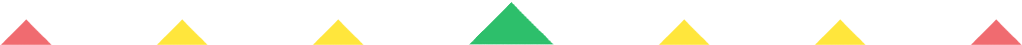Player 1
vs
Player 2
0:00
Opponent history
x
xCorrect
0
Wrong
0
Correct!
Wrong!
Help Sheet for

• 1 = I
• 2 = II
• 3 = III
• 4 = IV
• 5 = V
• 6 = VI
• 7 = VII
• 8 = VIII
• 9 = IX
• 10 = X

• 11 = XI
• 12 = XII
• 13 = XIII
• 14 = XIV
• 15 = XV
• 16 = XVI
• 17 = XVII
• 18 = XVIII
• 19 = XIX
• 20 = XX

• 21 = XXI
• 22 = XXII
• 23 = XXIII
• 24 = XXIV
• 25 = XXV
• 26 = XXVI
• 27 = XXVII
• 28 = XXVIII
• 29 = XXIX
• 30 = XXX

• 31 = XXXI
• 32 = XXXII
• 33 = XXXIII
• 34 = XXXIV
• 35 = XXXV
• 36 = XXXVI
• 37 = XXXVII
• 38 = XXXVIII
• 39 = XXXIX
• 40 = XL

• 41 = XLI
• 42 = XLII
• 43 = XLIII
• 44 = XLIV
• 45 = XLV
• 46 = XLVI
• 47 = XLVII
• 48 = XLVIII
• 49 = XLIX
• 50 = L

• 51 = LI
• 52 = LII
• 53 = LIII
• 54 = LIV
• 55 = LV
• 56 = LVI
• 57 = LVII
• 58 = LVIII
• 59 = LIX
• 60 = LX

• 61 = LXI
• 62 = LXII
• 63 = LXIII
• 64 = LXIV
• 65 = LXV
• 66 = LXVI
• 67 = LXVII
• 68 = LXVIII
• 69 = LXIX
• 70 = LXX

• 71 = LXXI
• 72 = LXXII
• 73 = LXXIII
• 74 = LXXIV
• 75 = LXXV
• 76 = LXXVI
• 77 = LXXVII
• 78 = LXXVIII
• 79 = LXXIX
• 80 = LXXX

###to 90

• 81 = LXXXI
• 82 = LXXXII
• 83 = LXXXIII
• 84 = LXXXIV
• 85 = LXXXV
• 86 = LXXXVI
• 87 = LXXXVII
• 88 = LXXXVIII
• 89 = LXXXIX
• 90 = XC

• 91 = XCI
• 92 = XCII
• 93 = XCIII
• 94 = XCIV
• 95 = XCV
• 96 = XCVI
• 97 = XCVII
• 98 = XCVIII
• 99 = XCIX
• 100 = C